##Involutive homographic relation III

Consider a circle bundle, for example the bundle (I) of circles (c) orthogonal to two fixed circles c1 and c2. Consider also an arbitrary circle d. For each point X on circle (d) there is a circle c(X) of bundle (I) passing through X. Let Y be the second intersection point of c(X) with (d). Use a point O of the circle (d) to define a good parametrization of the circle on the points of x-axis. In this parametrization we identify point X with the intersection point x of line OX with the x-axis. The correspondence y=F(x) is an involutive homography relation between x and y. The image shows the graph of this relation, which as expected is a rectangular hyperbola symmetric with respect to the first diagonal. The fixed points of the involution (if any, then two X1, X2) correspond to the circles of bundle (I) which are tangent to circle (d). On the graph they define its intersection points with the first diagonal.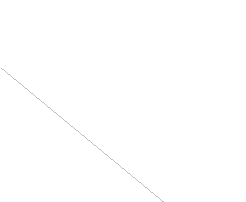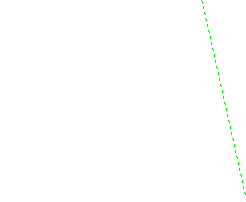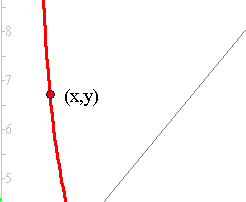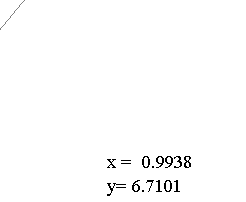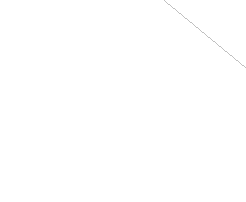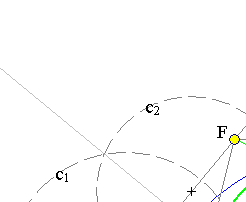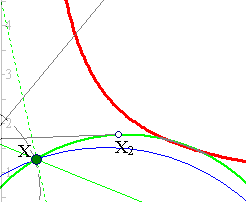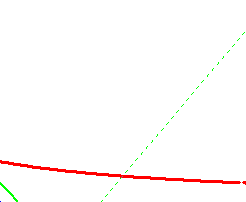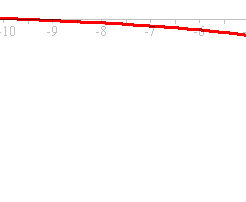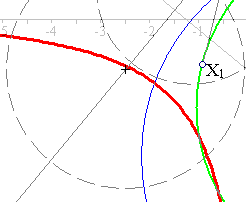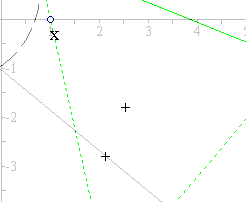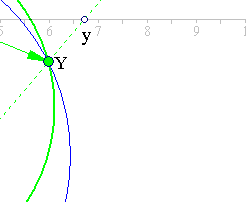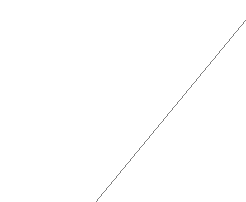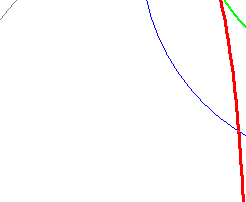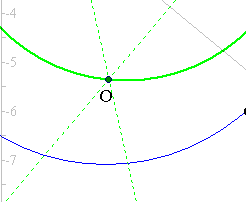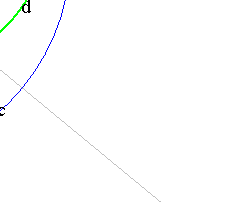Notice that all lines XY, coinciding with the radical axis of circles (c) and (d) pass through the same point F lying on the common radical axis of all circles (c). In fact F is the radical center of (d) and every pair of circles of the bundle (I). The fixed points X1, X2 of the involution are the contact points of the tangents from F. On circle (d) the involution coincides with the inversion with respect to the circle (F, |FX1|).

### See Also

CircleBundles.html
HomographicRelation.html
Involution.html
Involution2.html

 Produced with EucliDraw© http://users.math.uoc.gr/~pamfilos/